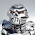## Monday, 2 April 2018

### Target Locked On Number Crunching

I tried to use the system in the Target Locked On aircraft building section to create stats for the JAS-39 Gripen yesterday, but ran into a problem. The MAN rating is easy to work out, based on the Thrust to Weight ratio x 5, which for the Gripen works out at 0.97 x 5 = 4.85, rounded up to 5. However, when calculating HITS the formula doesn't work, giving a Size Factor number that is way off the mark.

HITS are based on the Size Factor (SF) of the aircraft, which is worked out using this formula:

Length (M) + Wingspan (M) + Height (M) / 3

The HITS value is then worked out using the following calculation:

Size Factor x 10 + 1 (the end result should be a number between 0 and 1)

So, for the JAS-39C Gripen, based on the aircraft's dimensions, the numbers crunch like this:

Size Factor: 14.1 + 8.4 + 4.5 = 27 / 3 = 9

HITS: 9 x 10 + 1 = 91

This is obviously not the right answer and, therefore, screws up the other aircraft stats which are also based on the Size Factor including TARGET and FUEL. So, I played around with the formula to see if I could make it work and fit with the existing aircraft stats provided in the rules. The obvious thing to do is this:

Size Factor =  Length (M) + Wingspan (M) + Height (M) / 100 (not 3)

This gives the Gripen the following SF and HITS values:

14.1 + 8.4 + 4.5 = 27 / 100 = 0.27 (rounded down to) 0.2

HITS = 0.2 x 10 + 1 = 3

The TARGET and FUEL factors then work out as 5+ and 18 respectively, using the tables in the rules to calculate the numbers based on the Size Factor (SF). If I rounded the SF up to 0.3 the Gripen ends up the same size as an F-15 or F-4, which are larger and more powerful aircraft by far.

This gives the Gripen the same HITS, TARGET and almost the same FUEL factors as an F-16, which is the closest equivalent multi-role fighter aircraft. If the FUEL factor is fudged to a round 20, in line with all of the other aircraft in the lists, then it's spot on in comparison.

To double check, I used the same method to calculate the various stats for the Eurofighter Typhoon, which also worked out spot on apart from the FUEL rating of 18 not 20. Again, as all fighter aircraft in the listings have a FUEL rating of 20, this is easily sorted out by just rounding it up in line with all the other planes.

I will drop an email to Rory Crabb to see what he thinks about this and to check that I'm not barking up the wrong tree!

1.2.3.